Theory and Modern Applications

# Fractional complex transforms for fractional differential equations

## Abstract

The fractional complex transform is employed to convert fractional differential equations analytically in the sense of the Srivastava-Owa fractional operator and its generalization in the unit disk. Examples are illustrated to elucidate the solution procedure including the space-time fractional differential equation in complex domain, singular problems and Cauchy problems. Here, we consider analytic solutions in the complex domain.

MSC:30C45.

## 1 Introduction

The theory of fractional calculus has been applied in the theory of analytic functions. The classical concepts of a fractional differential operator and a fractional integral operator and their generalizations have fruitfully been employed in finding, for example, the characterization properties, coefficients estimate , distortion inequalities  and convolution properties for difference subclasses of analytic functions.

Fractional differential equations are viewed as alternative models to nonlinear differential equations. Varieties of them play important roles and serve as tools not only in mathematics, but also in physics, dynamical systems, control systems, and engineering to create the mathematical modeling of many physical phenomena. Furthermore, they are employed in social sciences such as food supplement, climate, and economics. Fractional differential equations concerning the Riemann-Liouville fractional operators or the Caputo derivative have been recommended by many authors (see ).

Recently, the complex modelings of phenomena in nature and society have been the object of several investigations based on the methods originally developed in a physical context. These systems are the consequence of the ability of individuals to develop strategies. They occur in kinetic theory , complex dynamical systems , chaotic complex systems and hyperchaotic complex systems , and the complex Lorenz-like system which has been found in laser physics while analyzing baroclinic instability of the geophysical flows in the atmosphere (or in the ocean) [14, 15]. Sainty  considered the complex heat equation using a complex valued Brownian. A model of complex fractional equations is introduced by Jumarie  using different types of fractional derivatives. Baleanu et al.  imposed several applications of fractional calculus including complex modelings. The author studied various types of fractional differential equations in complex domain such as the Cauchy equation, the diffusion equation and telegraph equations .

Transform is a significant technique to solve mathematical problems. Many useful transforms for solving various problems appeared in open literature such as wave transformation, the Laplace transform, the Fourier transform, the Bücklund transformation, the integral transform, the local fractional integral transforms and the fractional complex transform (see [29, 30]).

In this paper, we shall introduce two generalizations of the wave transformation in the complex domain. These generalizations depend on the fractional differential operators for complex variables. These transformations convert the fractional differential equations in complex domain into ordinary differential equations to obtain analytic solutions or exact solutions. Examples are illustrated.

In , Srivastava and Owa provided the definitions for fractional operators (derivative and integral) in the complex z-plane as follows.

Definition 1.1 The fractional derivative of order α is defined, for a function $f\left(z\right)$, by

${D}_{z}^{\alpha }f\left(z\right):=\frac{1}{\mathrm{\Gamma }\left(1-\alpha \right)}\frac{d}{dz}{\int }_{0}^{z}\frac{f\left(\zeta \right)}{{\left(z-\zeta \right)}^{\alpha }}\phantom{\rule{0.2em}{0ex}}d\zeta ;\phantom{\rule{1em}{0ex}}0\le \alpha <1,$

where the function $f\left(z\right)$ is analytic in a simply-connected region of the complex z-plane containing the origin, and the multiplicity of ${\left(z-\zeta \right)}^{-\alpha }$ is removed by requiring $log\left(z-\zeta \right)$ to be real when $\left(z-\zeta \right)>0$.

Definition 1.2 The fractional integral of order α is defined, for a function $f\left(z\right)$, by

${I}_{z}^{\alpha }f\left(z\right):=\frac{1}{\mathrm{\Gamma }\left(\alpha \right)}{\int }_{0}^{z}f\left(\zeta \right){\left(z-\zeta \right)}^{\alpha -1}\phantom{\rule{0.2em}{0ex}}d\zeta ;\phantom{\rule{1em}{0ex}}\alpha >0,$

where the function $f\left(z\right)$ is analytic in a simply-connected region of the complex z-plane $\left(\mathbb{C}\right)$ containing the origin, and the multiplicity of ${\left(z-\zeta \right)}^{\alpha -1}$ is removed by requiring $log\left(z-\zeta \right)$ to be real when $\left(z-\zeta \right)>0$.

Remark 1.1 From Definitions 1.1 and 1.2, we have

${D}_{z}^{\alpha }{z}^{\beta }=\frac{\mathrm{\Gamma }\left(\beta +1\right)}{\mathrm{\Gamma }\left(\beta -\alpha +1\right)}{z}^{\beta -\alpha },\phantom{\rule{1em}{0ex}}\beta >-1;0\le \alpha <1$

and

${I}_{z}^{\alpha }{z}^{\beta }=\frac{\mathrm{\Gamma }\left(\beta +1\right)}{\mathrm{\Gamma }\left(\beta +\alpha +1\right)}{z}^{\beta +\alpha },\phantom{\rule{1em}{0ex}}\beta >-1;\alpha >0.$

In  the author derived a formula for the generalized fractional integral. Consider for natural $n\in \mathbb{N}=\left\{1,2,\dots \right\}$ and real μ, the n-fold integral of the form

${I}_{z}^{\alpha ,\mu }f\left(z\right)={\int }_{0}^{z}{\zeta }_{1}^{\mu }\phantom{\rule{0.2em}{0ex}}d{\zeta }_{1}{\int }_{0}^{{\zeta }_{1}}{\zeta }_{2}^{\mu }\phantom{\rule{0.2em}{0ex}}d{\zeta }_{2}\cdots {\int }_{0}^{{\zeta }_{n-1}}{\zeta }_{n}^{\mu }f\left({\zeta }_{n}\right)\phantom{\rule{0.2em}{0ex}}d{\zeta }_{n},$
(1)

which yields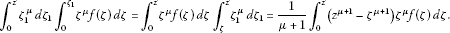Repeating the above step $n-1$ times, we obtain

${\int }_{0}^{z}{\zeta }_{1}^{\mu }\phantom{\rule{0.2em}{0ex}}d{\zeta }_{1}{\int }_{0}^{{\zeta }_{1}}{\zeta }_{2}^{\mu }\phantom{\rule{0.2em}{0ex}}d{\zeta }_{2}\cdots {\int }_{0}^{{\zeta }_{n-1}}{\zeta }_{n}^{\mu }f\left({\zeta }_{n}\right)\phantom{\rule{0.2em}{0ex}}d{\zeta }_{n}=\frac{{\left(\mu +1\right)}^{1-n}}{\left(n-1\right)!}{\int }_{0}^{z}{\left({z}^{\mu +1}-{\zeta }^{\mu +1}\right)}^{n-1}{\zeta }^{\mu }f\left(\zeta \right)\phantom{\rule{0.2em}{0ex}}d\zeta ,$

which implies the fractional operator type

${I}_{z}^{\alpha ,\mu }f\left(z\right)=\frac{{\left(\mu +1\right)}^{1-\alpha }}{\mathrm{\Gamma }\left(\alpha \right)}{\int }_{0}^{z}{\left({z}^{\mu +1}-{\zeta }^{\mu +1}\right)}^{\alpha -1}{\zeta }^{\mu }f\left(\zeta \right)\phantom{\rule{0.2em}{0ex}}d\zeta ,$
(2)

where a and $\mu \ne -1$ are real numbers and the function $f\left(z\right)$ is analytic in a simply-connected region of the complex z-plane containing the origin, and the multiplicity of ${\left({z}^{\mu +1}-{\zeta }^{\mu +1}\right)}^{-\alpha }$ is removed by requiring $log\left({z}^{\mu +1}-{\zeta }^{\mu +1}\right)$ to be real when $\left({z}^{\mu +1}-{\zeta }^{\mu +1}\right)>0$. When $\mu =0$, we get the standard Srivastava-Owa fractional integral, which is applied to define the Srivastava-Owa fractional derivatives. The computation implies 

${I}_{z}^{\alpha ,\mu }{z}^{\nu }=\frac{{z}^{\alpha \left(\mu +1\right)+\nu }}{{\left(\mu +1\right)}^{\alpha }}\frac{\mathrm{\Gamma }\left(\frac{\nu +\mu +1}{\mu +1}\right)}{\mathrm{\Gamma }\left(\alpha +\frac{\nu +\mu +1}{\mu +1}\right)}.$
(3)

When $\mu =0$, we obtain ${I}_{z}^{\alpha }{z}^{\nu }=\frac{\mathrm{\Gamma }\left(\nu +1\right)}{\mathrm{\Gamma }\left(\alpha +\nu +1\right)}{z}^{\alpha +\nu }$ (see Remark 1.1).

Corresponding to the generalized fractional integrals (2), we imposed the generalized differential operator.

Definition 1.3 The generalized fractional derivative of order α is defined, for a function $f\left(z\right)$, by

${D}_{z}^{\alpha ,\mu }f\left(z\right):=\frac{{\left(\mu +1\right)}^{\alpha }}{\mathrm{\Gamma }\left(1-\alpha \right)}\frac{d}{dz}{\int }_{0}^{z}\frac{{\zeta }^{\mu }f\left(\zeta \right)}{{\left({z}^{\mu +1}-{\zeta }^{\mu +1}\right)}^{\alpha }}\phantom{\rule{0.2em}{0ex}}d\zeta ;\phantom{\rule{1em}{0ex}}0\le \alpha <1,$
(4)

where the function $f\left(z\right)$ is analytic in a simply-connected region of the complex z-plane containing the origin, and the multiplicity of ${\left({z}^{\mu +1}-{\zeta }^{\mu +1}\right)}^{-\alpha }$ is removed by requiring $log\left({z}^{\mu +1}-{\zeta }^{\mu +1}\right)$ to be real when $\left({z}^{\mu +1}-{\zeta }^{\mu +1}\right)>0$. The calculation yields

${D}_{z}^{\alpha ,\mu }{z}^{\nu }=\frac{{\left(\mu +1\right)}^{\alpha -1}\mathrm{\Gamma }\left(\frac{\nu }{\mu +1}+1\right)}{\mathrm{\Gamma }\left(\frac{\nu }{\mu +1}+1-\alpha \right)}{z}^{\left(1-\alpha \right)\left(\mu +1\right)+\nu -1}.$
(5)

When $\mu =0$, we obtain ${D}_{z}^{\alpha }{z}^{\nu }=\frac{\mathrm{\Gamma }\left(\nu +1\right)}{\mathrm{\Gamma }\left(\nu +1-\alpha \right)}{z}^{\nu -\alpha }$ (see Remark 1.1).

For analytic functions of the form

$f\left(z\right)=\sum _{n=1}^{\mathrm{\infty }}{a}_{n}{z}^{n},\phantom{\rule{1em}{0ex}}z\in U,$
(6)

we have the following property:

${D}_{z}^{\alpha ,\mu }{I}_{z}^{\alpha ,\mu }f\left(z\right)={I}_{z}^{\alpha ,\mu }{D}_{z}^{\alpha ,\mu }f\left(z\right)=f\left(z\right),\phantom{\rule{1em}{0ex}}z\in U.$

## 2 Fractional complex transform

In recent times, one of the most important and useful methods for fractional calculus called fractional complex transform has appeared . Fractional complex transform is to renovate the fractional differential equations into ordinary differential equations, yielding a tremendously simple solution procedure. In this section, we illustrate some fractional complex transform using properties of the Srivastava-Owa fractional operator and its generalization. Analogous to wave transformation

$\eta =az+bw+cu+\cdots ,$
(7)

where a, b, and c are constants, the fractional complex transform is

$\eta =a{z}^{\alpha }+b{w}^{\beta }+c{u}^{\gamma }+\cdots$
(8)

for the fractional differential equations in the sense of the Srivastava-Owa fractional operators. While the fractional complex transform of the form

$\eta =A{z}^{\alpha \left(\mu +1\right)}+B{w}^{\beta \left(\mu +1\right)}+C{u}^{\gamma \left(\mu +1\right)}+\cdots$
(9)

is applied to fractional differential equations in the sense of the generalized operators (2) and (4). It is obvious that when $\mu =0$, (9) reduces to (8). Furthermore, in a real case, (9) implies the fractional complex transform defined in . We impose the fractional complex transform

${D}_{z}^{\alpha }f\left(z\right)=\frac{\partial f}{\partial Z}{D}_{z}^{\alpha }Z,\phantom{\rule{1em}{0ex}}Z:={z}^{\alpha }$
(10)

if we denote ${D}_{z}^{\alpha }f\left(z\right):=\frac{{\partial }^{\alpha }f}{\partial {z}^{\alpha }}$, it yields

$\begin{array}{rcl}\frac{{\partial }^{\alpha }f}{\partial {z}^{\alpha }}& =& \frac{\partial f}{\partial Z}\frac{{\partial }^{\alpha }Z}{\partial {z}^{\alpha }}\\ :=& \frac{\partial f}{\partial Z}{\theta }_{\alpha },\end{array}$
(11)

where ${\theta }_{\alpha }$ is the fractal index, which is usually determined in terms of gamma functions. Similarly, we can receive

${D}_{z}^{\alpha ,\mu }f\left(z\right)=\frac{\partial f}{\partial Z}{D}_{z}^{\alpha ,\mu }Z,\phantom{\rule{1em}{0ex}}Z:={z}^{\alpha \left(\mu +1\right)}$
(12)

if we let ${D}_{z}^{\alpha ,\mu }f\left(z\right):=\frac{{\partial }^{\alpha \left(\mu +1\right)}f}{\partial {z}^{\alpha \left(\mu +1\right)}}$, which implies

$\begin{array}{rcl}\frac{{\partial }^{\alpha \left(\mu +1\right)}f}{\partial {z}^{\alpha \left(\mu +1\right)}}& =& \frac{\partial f}{\partial Z}\frac{{\partial }^{\alpha \left(\mu +1\right)}Z}{\partial {z}^{\alpha \left(\mu +1\right)}}\\ :=& \frac{\partial f}{\partial Z}{\mathrm{\Theta }}_{\alpha ,\mu }.\end{array}$
(13)

Example 2.1 Let $Z={z}^{\alpha }$ and $f={Z}^{n}$, $n\ne 0$ then in view of Remark 1.1, we obtain

$\begin{array}{rcl}\frac{{\partial }^{\alpha }f}{\partial {z}^{\alpha }}& =& \frac{\partial f}{\partial Z}\frac{{\partial }^{\alpha }Z}{\partial {z}^{\alpha }}\\ =& \frac{\mathrm{\Gamma }\left(1+n\alpha \right){z}^{n\alpha -\alpha }}{\mathrm{\Gamma }\left(1+n\alpha -\alpha \right)}\\ :=& \frac{\partial f}{\partial Z}{\theta }_{\alpha }\\ =& n{\theta }_{\alpha }{z}^{n\alpha -\alpha }.\end{array}$

${\theta }_{\alpha }=\frac{\mathrm{\Gamma }\left(1+n\alpha \right)}{n\mathrm{\Gamma }\left(1+n\alpha -\alpha \right)}.$

Example 2.2 Let $Z={z}^{\alpha \left(\mu +1\right)}$ and $f={Z}^{\frac{n}{\mu +1}}$, $n\ne 0$ then in virtue of (5), we have

$\begin{array}{rcl}\frac{{\partial }^{\alpha \left(\mu +1\right)}f}{\partial {z}^{\alpha \left(\mu +1\right)}}& =& \frac{\partial f}{\partial Z}\frac{{\partial }^{\alpha \left(\mu +1\right)}{Z}^{n}}{\partial {z}^{\alpha \left(\mu +1\right)}}\\ =& \frac{{\left(\mu +1\right)}^{\alpha -1}\mathrm{\Gamma }\left(\frac{n\alpha }{\mu +1}+1\right)}{\mathrm{\Gamma }\left(\frac{n\alpha }{\mu +1}+1-\alpha \right)}{z}^{\left(1-\alpha \right)\left(\mu +1\right)+n\alpha -1}\\ :=& \frac{\partial f}{\partial Z}{\mathrm{\Theta }}_{\alpha ,\mu }\\ =& \frac{n{\mathrm{\Theta }}_{\alpha ,\mu }}{\mu +1}{z}^{\left(1-\alpha \right)\left(\mu +1\right)+n\alpha -1}.\end{array}$

We, therefore, have

${\mathrm{\Theta }}_{\alpha ,\mu }=\frac{{\left(\mu +1\right)}^{\alpha }\mathrm{\Gamma }\left(\frac{n\alpha }{\mu +1}+1\right)}{n\mathrm{\Gamma }\left(\frac{n\alpha }{\mu +1}+1-\alpha \right)}.$

## 3 Applications

Example 3.1 Consider the following equation:

(14)

where $u\left(t,z\right)$ is the unknown function $\rho \in \left(0,1\right)$ and $\beta \in \left(0,1\right]$.

We propose to show that (14) has a unique analytic solution by using the Banach fixed point theorem. By assuming

$u\left(t,z\right)=\mu \left(z\right)t+v\left(t,z\right)\phantom{\rule{1em}{0ex}}\left(v\left(t,z\right)=O\left({t}^{2}\right)\right)$

as a formal solution, where $\mu \left(z\right)=O\left({z}^{\beta }\right)$, calculations imply

${t}^{1/2}\frac{{\partial }^{1/2}u\left(t,z\right)}{\partial {t}^{1/2}}=1.128\mu \left(z\right)t+{t}^{1/2}{v}_{\alpha }\left(t,z\right),\phantom{\rule{1em}{0ex}}\alpha =1/2,$

and

${D}_{z}^{\beta }u\left(t,z\right)={D}_{z}^{\beta }\left(\mu \left(z\right)t+v\left(t,z\right)\right)=t{D}_{z}^{\beta }\mu \left(z\right)+{v}_{\beta }\left(t,z\right).$

Therefore, $\mu \left(z\right)$ satisfies

$\rho \mu \left(z\right)+{D}_{z}^{\beta }\mu \left(z\right)=0,$

which is equivalent to

${D}_{z}^{\beta }\mu \left(z\right)=g\left(z,\mu \left(z\right)\right),$
(15)

where

$g\left(z,\mu \left(z\right)\right)=-\rho \mu \left(z\right).$

Now $g\left(z,\mu \left(z\right)\right)$ is a contraction mapping whenever $\rho \in \left(0,1\right)$; therefore, in view of the Banach fixed point theorem, Eq. (15) has a unique analytic solution in the unit disk and consequently the problem (14).

To calculate the fractal index for the equation

${D}_{z}^{\beta }\mu \left(z\right)+\rho \mu \left(z\right)=0,\phantom{\rule{1em}{0ex}}\mu \left(0\right)=1,$
(16)

we assume the transform $Z={z}^{\beta }$ and the solution can be expressed in a series in the form

$\mu \left(Z\right)=\sum _{m=0}^{\mathrm{\infty }}{\mu }_{m}{Z}^{m},$
(17)

where ${\mu }_{m}$ are constants. Substituting (17) into Eq. (16) yields

$\frac{\partial }{\partial Z}\sum _{m=0}^{\mathrm{\infty }}{\theta }_{\beta m}{\mu }_{m}{Z}^{m}+\rho \sum _{m=0}^{\mathrm{\infty }}{\mu }_{m}{Z}^{m}=0.$
(18)

Since

${\theta }_{\beta m}=\frac{\mathrm{\Gamma }\left(1+m\beta \right)}{m\mathrm{\Gamma }\left(1+m\beta -\beta \right)},$

then the computation imposes the relation

$\frac{\mathrm{\Gamma }\left(1+m\beta \right)}{\mathrm{\Gamma }\left(1+m\beta -\beta \right)}{\mu }_{m}+\rho {\mu }_{m-1}=0$

with $\mu \left(0\right)=1$, and consequently we obtain

${\mu }_{m}=\frac{{\left(-\rho \right)}^{m}}{\mathrm{\Gamma }\left(1+m\beta \right)}.$

Thus, we have the following solution:

$\mu \left(Z\right)=\sum _{m=0}^{\mathrm{\infty }}\frac{{\left(-\rho \right)}^{m}}{\mathrm{\Gamma }\left(1+m\beta \right)}{Z}^{m}$

which is equivalent to

$\mu \left(z\right)=\sum _{m=0}^{\mathrm{\infty }}\frac{{\left(-\rho \right)}^{m}}{\mathrm{\Gamma }\left(1+m\beta \right)}{z}^{m\beta }={E}_{\beta }\left(-\rho {z}^{\beta }\right),$

where ${E}_{\beta }$ is a Mittag-Leffler function. The last assertion is the exact solution for the problem (16) and consequently for (14).

Example 3.2 Consider the following equation:

(19)

where $u\left(t,z\right)$ is the unknown function and $\beta \in \left(0,1\right]$. In the same manner of Example 3.1, we let

$u\left(t,z\right)=\mu \left(z\right)t+v\left(t,z\right)\phantom{\rule{1em}{0ex}}\left(v\left(t,z\right)=O\left({t}^{2}\right)\right)$

as a formal solution, where $\mu \left(z\right)=O\left({z}^{\beta }\right)$ and

$|{\mu }^{\prime }\left(z\right)-{\nu }^{\prime }\left(z\right)|<\lambda |\mu \left(z\right)-\nu \left(z\right)|,\phantom{\rule{1em}{0ex}}\lambda <\frac{1}{8}.$

Estimations imply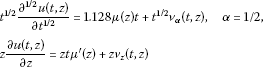and

${D}_{z}^{\beta }u\left(t,z\right)={D}_{z}^{\beta }\left(\mu \left(z\right)t+v\left(t,z\right)\right)=t{D}_{z}^{\beta }\mu \left(z\right)+{v}_{\beta }\left(t,z\right).$

Therefore, $\mu \left(z\right)$ satisfies

$\frac{\mu \left(z\right)}{2}+4z{\mu }^{\prime }\left(z\right)+{D}_{z}^{\beta }\mu \left(z\right)-{z}^{\beta }=0,$

which is equivalent to

${D}_{z}^{\beta }\mu \left(z\right)=G\left(z,\mu \left(z\right),z{\mu }^{\prime }\left(z\right)\right),$
(20)

where

$G\left(z,\mu \left(z\right),z{\mu }^{\prime }\left(z\right)\right)={z}^{\beta }-1/2\mu \left(z\right)-4z{\mu }^{\prime }\left(z\right).$

Now, to show that $G\left(z,\mu \left(z\right),z{\mu }^{\prime }\left(z\right)\right)$ is a contraction mapping,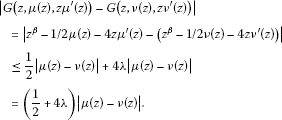Thus, in view of the Banach fixed point theorem, Eq. (20) has a unique analytic solution in the unit disk and consequently the problem (19).

To evaluate the fractal index for the equation

${D}_{z}^{\beta }\mu \left(z\right)+\frac{\mu \left(z\right)}{2}+4z{\mu }^{\prime }\left(z\right)-{z}^{\beta }=0,\phantom{\rule{1em}{0ex}}\mu \left(0\right)=1,$
(21)

we assume the transform $Z={z}^{\beta }$ and the solution can be articulated as in (17). Substituting (17) into Eq. (21), we have

$\frac{\partial }{\partial Z}\sum _{m=0}^{\mathrm{\infty }}{\theta }_{\beta m}{\mu }_{m}{Z}^{m}+\frac{1}{2}\sum _{m=0}^{\mathrm{\infty }}{\mu }_{m}{Z}^{m}+4\sum _{m=1}^{\mathrm{\infty }}m{\mu }_{m}{Z}^{m}-Z=0,$
(22)

where

${\theta }_{\beta m}=\frac{\mathrm{\Gamma }\left(1+m\beta \right)}{m\mathrm{\Gamma }\left(1+m\beta -\beta \right)}.$

Hence, the computation imposes the relation

$\left(\frac{\mathrm{\Gamma }\left(1+m\beta \right)}{\mathrm{\Gamma }\left(1+m\beta -\beta \right)}+4m\right){\mu }_{m}+\frac{1}{2}{\mu }_{m-1}=0$

with $\mu \left(0\right)=1$, and consequently we obtain

${\mu }_{m}:=\frac{{\left({B}_{m}\right)}^{m}}{\mathrm{\Gamma }\left(1+m\beta \right)},$

where ${B}_{m}$ in terms of a gamma function. If we let $B:={max}_{m}\left\{{B}_{m}\right\}$, then the solution approximates to

$\mu \left(Z\right)\simeq \sum _{m=0}^{\mathrm{\infty }}\frac{{\left(B\right)}^{m}}{\mathrm{\Gamma }\left(1+m\beta \right)}{Z}^{m},$

which is equivalent to

$\mu \left(z\right)=\sum _{m=0}^{\mathrm{\infty }}\frac{{\left(B\right)}^{m}}{\mathrm{\Gamma }\left(1+m\beta \right)}{z}^{m\beta }={E}_{\beta }\left(B{z}^{\beta }\right).$

The last assertion is the exact solution for the problem (21) and consequently for (19).

Next, we consider the Cauchy problem by employing the generalized fractional differential operator (4). We shall show that the solution of such a problem can be determined in terms of the Fox-Wright function :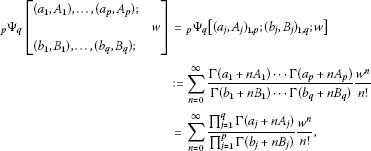where ${A}_{j}>0$ for all $j=1,\dots ,p$, ${B}_{j}>0$ for all $j=1,\dots ,q$, and $1+{\sum }_{j=1}^{q}{B}_{j}-{\sum }_{j=1}^{p}{A}_{j}\ge 0$ for suitable values $|w|<1$ and ${a}_{i}$, ${b}_{j}$ are complex parameters.

Example 3.3 Consider the Cauchy problem in terms of the differential operator (4)

${D}_{z}^{\alpha ,\mu }u\left(z\right)=F\left(z,u\left(z\right)\right),$
(23)

where $F\left(z,u\left(z\right)\right)$ is analytic in u and $u\left(z\right)$ is analytic in the unit disk. Thus, F can be expressed by

$F\left(z,u\right)=\varphi u\left(z\right).$

Let $Z={z}^{\alpha \left(\mu +1\right)}$. Then the solution can be formulated as follows:

$u\left(Z\right)=\sum _{m=0}^{\mathrm{\infty }}{u}_{m}{Z}^{m},$
(24)

where ${u}_{m}$ are constants. Substituting (24) into Eq. (23) implies

$\frac{\partial }{\partial Z}\sum _{m=0}^{\mathrm{\infty }}{\mathrm{\Theta }}_{\alpha ,\mu ,m}{u}_{m}{Z}^{m}-\varphi \sum _{m=0}^{\mathrm{\infty }}{u}_{m}{Z}^{m}=0.$
(25)

Since

${\mathrm{\Theta }}_{\alpha ,\mu ,m}=\frac{{\left(\mu +1\right)}^{\alpha }\mathrm{\Gamma }\left(\frac{m\alpha }{\mu +1}+1\right)}{m\mathrm{\Gamma }\left(\frac{n\alpha }{\mu +1}+1-\alpha \right)},$

then the calculation yields the relation

$\frac{{\left(\mu +1\right)}^{\alpha }\mathrm{\Gamma }\left(\frac{m\alpha }{\mu +1}+1\right)}{\mathrm{\Gamma }\left(\frac{m\alpha }{\mu +1}+1-\alpha \right)}{u}_{m}-\varphi {u}_{m-1}=0;$

consequently, we obtain

${u}_{m}={\left[\frac{\varphi }{{\left(\mu +1\right)}^{\alpha }}\right]}^{m}\frac{\mathrm{\Gamma }\left(\frac{\left(m-1\right)\alpha }{\mu +1}+1-\alpha \right)\mathrm{\Gamma }\left(\frac{m\alpha }{\mu +1}+1-\alpha \right)}{\mathrm{\Gamma }\left(\frac{\left(m-1\right)\alpha }{\mu +1}+1\right)\mathrm{\Gamma }\left(\frac{m\alpha }{\mu +1}+1\right)}.$

Thus, we have the following solution:

$u\left(Z\right)=\sum _{m=0}^{\mathrm{\infty }}{\left[\frac{\varphi }{{\left(\mu +1\right)}^{\alpha }}\right]}^{m}\frac{\mathrm{\Gamma }\left(\frac{\left(m-1\right)\alpha }{\mu +1}+1-\alpha \right)\mathrm{\Gamma }\left(\frac{m\alpha }{\mu +1}+1-\alpha \right)}{\mathrm{\Gamma }\left(\frac{\left(m-1\right)\alpha }{\mu +1}+1\right)\mathrm{\Gamma }\left(\frac{m\alpha }{\mu +1}+1\right)}{Z}^{m}$

which is equivalent to

$u\left(Z\right)=\sum _{m=0}^{\mathrm{\infty }}{\left[\frac{\varphi }{{\left(\mu +1\right)}^{\alpha }}\right]}^{m}\frac{\mathrm{\Gamma }\left(m+1\right)\mathrm{\Gamma }\left(\frac{\left(m-1\right)\alpha }{\mu +1}+1-\alpha \right)\mathrm{\Gamma }\left(\frac{m\alpha }{\mu +1}+1-\alpha \right)}{\mathrm{\Gamma }\left(\frac{\left(m-1\right)\alpha }{\mu +1}+1\right)\mathrm{\Gamma }\left(\frac{m\alpha }{\mu +1}+1\right)}\frac{{Z}^{m}}{m!}.$

Since ϕ is an arbitrary constant, we assume that

$\varphi :={\left(\mu +1\right)}^{\alpha }.$

Thus, for a suitable α, we present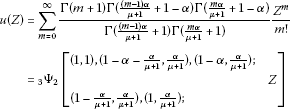or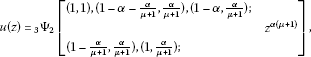where $|z|<1$.

## 4 Conclusion

A generalized fractional complex transform is suggested in this paper to find exact solutions of fractional differential equations in the unit disk. We have converted some classes of fractional differential equations in the sense of the Srivastava-Owa fractional operator and its generalization into ordinary differential equations. Hence, the exact solutions are imposed. The solution procedure is simple and might find wide applications in image processing and signal processing by using fractional filter mask. Examples 3.1 and 3.2 showed the conversion of time-space fractional differential equations into a normal case. The exact solutions are introduced in the expression of a Mittag-Leffler function. While Example 3.3, the Cauchy problem of fractional order in the unit disk, proposed the exact solution in terms of the Fox-Wright function.

## References

1. Darus M, Ibrahim RW: Radius estimates of a subclass of univalent functions. Math. Vesnik 2011, 63: 55–58.

2. Srivastava HM, Ling Y, Bao G: Some distortion inequalities associated with the fractional derivatives of analytic and univalent functions. JIPAM. J. Inequal. Pure Appl. Math. 2001, 2: 1–6.

3. Miller KS, Ross B: An Introduction to the Fractional Calculus and Fractional Differential Equations. Wiley, New York; 1993.

4. Podlubny I Mathematics in Science and Engineering 198. In Fractional Differential Equations. Academic Press, San Diego; 1999.

5. Hilfer R: Applications of Fractional Calculus in Physics. World Scientific Publishing, River Edge; 2000.

6. West BJ, Bologna M, Grigolini P Institute for Nonlinear Science. In Physics of Fractal Operators. Springer, New York; 2003.

7. Kilbas AA, Srivastava HM, Trujillo JJ North-Holland Mathematics Studies 204. In Theory and Applications of Fractional Differential Equations. Elsevier, Amsterdam; 2006.

8. Sabatier J, Agrawal OP, Machado JA: Advance in Fractional Calculus: Theoretical Developments and Applications in Physics and Engineering. Springer, Dordrecht; 2007.

9. Baleanu D, Guvenc B, Tenreiro JA: New Trends in Nanotechnology and Fractional Calculus Applications. Springer, New York; 2010.

10. Lakshmikantham V, Leela S, Vasundhara J: Theory of Fractional Dynamic Systems. Cambridge Academic Publishers, Cambridge; 2009.

11. Bianca C: On set of nonlinearity in thermostatted active particles models for complex systems. Nonlinear Anal., Real World Appl. 2012, 13: 2593–2608. 10.1016/j.nonrwa.2012.03.005

12. Mahmoud G, Mahmoud E: Modified projective Lag synchronization of two nonidentical hyperchaotic complex nonlinear systems. Int. J. Bifurc. Chaos 2011, 2(8):2369–2379.

13. Mahmoud G, Mahmoud E, Ahmed M: On the hyperchaotic complex Lü system. Nonlinear Dyn. 2009, 58: 725–738. 10.1007/s11071-009-9513-0

14. Fowler AC, Gibbon JD, McGuinness MJ: The complex Lorenz equations. Physica D 1982, 4: 139–163. 10.1016/0167-2789(82)90057-4

15. Rauth A, Hannibal L, Abraham NB: Global stability properties of the complex Lorenz model. Physica D 1996, 99: 45–58. 10.1016/S0167-2789(96)00129-7

16. Sainty P: Contraction of a complex-valued fractional Brownian of order n . J. Math. Phys. 1992, 33(9):3128–3149. 10.1063/1.529976

17. Jumarie G: Fractional Brownian motion with complex variance via random walk in the complex plane and applications. Chaos Solitons Fractals 2000, 11: 1097–1111. 10.1016/S0960-0779(99)00015-6

18. Jumarie G: Schrodinger equation for quantum fractal space-time of order n via the complex-valued fractional Brownian motion. Int. J. Mod. Phys. A 2001, 16: 5061–5084. 10.1142/S0217751X01005468

19. Jumarie G: Fractional Brownian motions via random walk in the complex plane and via fractional derivative. Comparison and further results. Chaos Solitons Fractals 2004, 22: 907–925. 10.1016/j.chaos.2004.03.020

20. Jumarie G: Fractionalization of the complex-valued Brownian motion of order n using Riemann-Liouville derivative. Applications to mathematical finance and stochastic mechanics. Chaos Solitons Fractals 2006, 28: 1285–1305. 10.1016/j.chaos.2005.08.083

21. Baleanu D, Diethelm K, Scalas E, Trujillo JJ: Fractional Calculus Models and Numerical Methods (Series on Complexity, Nonlinearity and Chaos). World Scientific, Singapore; 2012.

22. Baleanu D, Trujillo JJ: On exact solutions of a class of fractional Euler-Lagrange equations. Nonlinear Dyn. 2008, 52: 331–335. 10.1007/s11071-007-9281-7

23. Magin R, Feng X, Baleanu D: Solving the fractional order Bloch equation. Concepts Magn. Reson., Part A 2009, 34A: 16–23. 10.1002/cmr.a.20129

24. Ibrahim RW, Darus M: Subordination and superordination for univalent solutions for fractional differential equations. J. Math. Anal. Appl. 2008, 345: 871–879. 10.1016/j.jmaa.2008.05.017

25. Ibrahim RW: On solutions for fractional diffusion problems. Electron. J. Differ. Equ. 2010, 147: 1–11.

26. Ibrahim RW: Existence and uniqueness of holomorphic solutions for fractional Cauchy problem. J. Math. Anal. Appl. 2011, 380: 232–240. 10.1016/j.jmaa.2011.03.001

27. Ibrahim RW: Ulam stability for fractional differential equation in complex domain. Abstr. Appl. Anal. 2012., 2012: Article ID 649517. doi:10.1155/2012/649517

28. Ibrahim RW: On holomorphic solution for space and time fractional telegraph equations in complex domain. J. Funct. Spaces Appl. 2012., 2012: Article ID 703681. doi:10.1155/2012/703681

29. Gordoa PR, Pickering A, Zhu ZN: Bücklund transformations for a matrix second Painlev equation. Phys. Lett. A 2010, 374(34):3422–3424. 10.1016/j.physleta.2010.06.034

30. Molliq R, Batiha B: Approximate analytic solutions of fractional Zakharov-Kuznetsov equations by fractional complex transform. Int. J. Eng. Technol. 2012, 1(1):1–13.

31. Srivastava HM, Owa S: Univalent Functions, Fractional Calculus, and Their Applications. Wiley, New York; 1989.

32. Ibrahim RW: On generalized Srivastava-Owa fractional operators in the unit disk. Adv. Differ. Equ. 2011, 55: 1–10.

33. Li ZB, He JH: Fractional complex transform for fractional differential equations. Math. Comput. Appl. 2010, 15: 970–973.

34. Li ZB: An extended fractional complex transform. Int. J. Nonlinear Sci. Numer. Simul. 2010, 11: 0335–0337.

35. Li ZB, He JH: Application of the fractional complex transform to fractional differential equations. Nonlinear Sci. Lett. A 2011, 2: 121–126.

36. He J-H, Elagan SK, Li ZB: Geometrical explanation of the fractional complex transform and derivative chain rule for fractional calculus. Phys. Lett. A 2012, 376: 257–259. 10.1016/j.physleta.2011.11.030

37. Srivastava HM, Karlsson PW: Multiple Gaussian Hypergeometric Series. Wiley, New York; 1985.

## Author information

Authors

### Corresponding author

Correspondence to Rabha W Ibrahim.

### Competing interests

The author declares that they have no competing interests.

## Rights and permissions

Reprints and Permissions

Ibrahim, R.W. Fractional complex transforms for fractional differential equations. Adv Differ Equ 2012, 192 (2012). https://doi.org/10.1186/1687-1847-2012-192